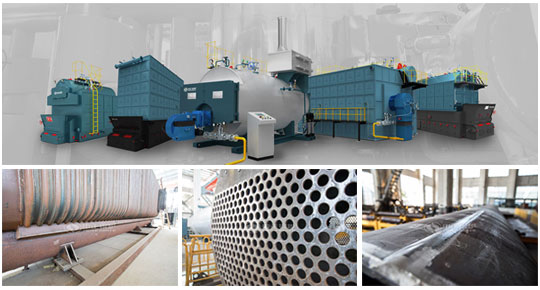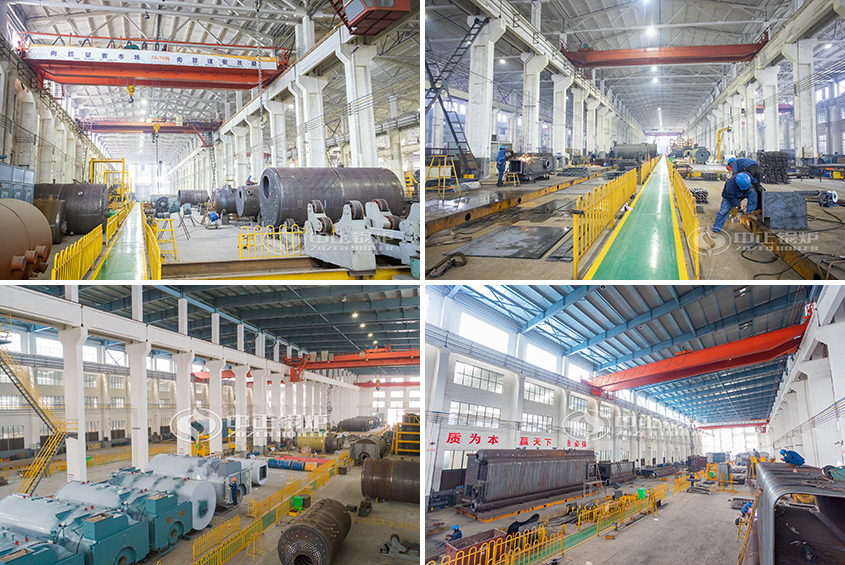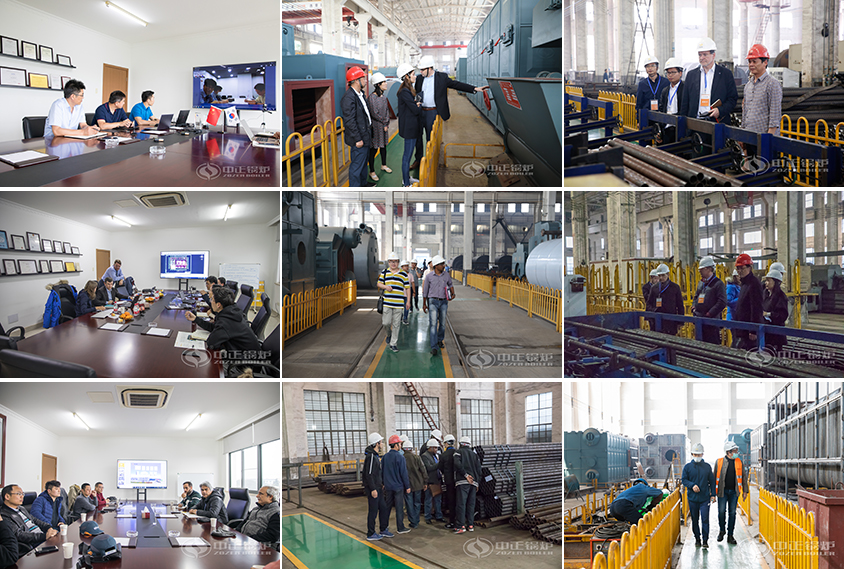# Boiler KW to Ton

August 6, 2020

The power (or output) of the boiler is the heat generated by the boiler per hour. The power of the hot water boiler is expressed in MW (1MW=1000kW) or ten thousand kcal/h (ten thousand kcal/h).
The power of the steam boiler is also called the evaporation capacity, which is the amount of water that turns into steam per hour: ton/hour (T/h) or kilogram/hour (kg/h). Of course, it can also be expressed in MW or kW.The corresponding relationship between evaporation and power is: 1T/h=1000kg/h=0.7MW=720kW=600,000 kcal/h=600Mcal/h.
The unit of power is horsepower (Hp) and boiler horsepower (BHp).
1Hp = 0.745kw, 1BHp = 9.81kw

European and American steam boiler evaporation capacity labels are often marked with the word “at 212”, which means that the evaporation capacity refers to the amount of steam that 212°F evaporates to 212°F? That is, 100°C water evaporates to 100°C steam. In this way, 1kg of evaporation is equivalent to 540kcal heat, we call it “equivalent evaporation”, namely: 1T/h = 540,000 kcal/h. It can also be calculated from this that the relationship between boiler horsepower and “equivalent evaporation” is: 1BHp = 15.62kg/h.

1. Boiler evaporation and boiler thermal efficiency
1 ton/hour (t/h) ≈60×104 kcal (kcal)/hour (kcal/h) ≈0.7 megawatt (MW) ≈720K kilowatt (KW)
2. Boiler evaporation and boiler horsepower 1 ton/hour (t/h)≈71.1 boiler horsepower (BHP)
3. Boiler pressure engineering unit and international unit of measurement 1 megapascal (Mpa)≈10 kgf/cm2 (kgf/cm2)
4. Megapascals and Pascals 1 megapascal (Mpa) = 106 Pa (pa) 1 Pa (pa) = 0.01mbar (mbar) ≈ 10-5 kgf/cm2 (engineering atmospheric pressure) (kgf/cm2) 1 Pa (pa)≈0.1 mm water column (mmH2O)
5. Force and gravity 1 kilogram force (kgf) = 9.81 Newton (N)
6. Calories 1 kcal (kcal) (kcal) = 4.187 kilojoules (KJ)
7. Body (volume) volume 1 cubic meter (m3) = 1000 liters (L) 1 liter (L) = 1000 milliliters (ML)

## Boiler Production Workshop## Customer Visiting